##### Page tree
Skip to end of banner
Go to start of banner

# KBSW180148 Instruction for Integrating SLAMWARE Solution in Tri-omini-wheeled Base

Skip to end of metadata
Go to start of metadata

# 底盘类型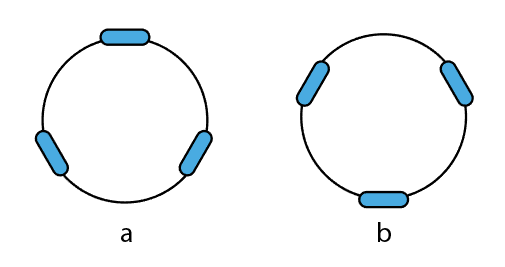# 定义

## 机器人坐标系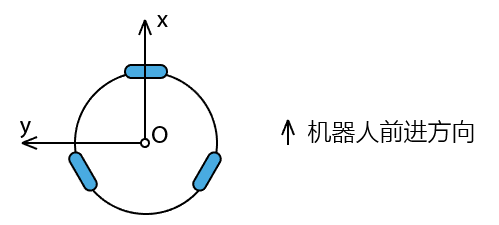## 机器人运动控制指令

SLAMWARE Core模块采用统一的全向速度指令来对机器人进行运动控制，其坐标系如下：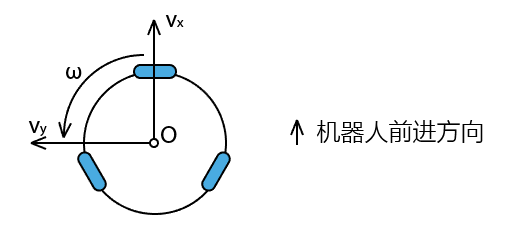## 轮组速度定义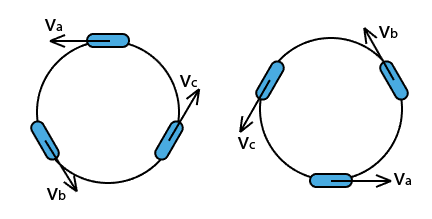Va，Vb和Vc分别表示各个轮子的线速度，以让机器人发生逆时针旋转的方向为正，单位依然是米每秒

## 机器人位移定义

SLAMWARE在向底盘下达运动指令的同时，需要底盘上报机器人轮组的位移信息。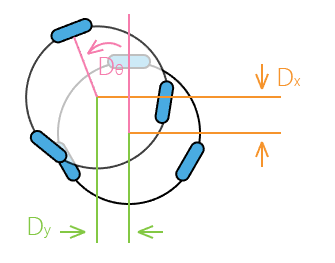# 涉及指令

base_set_velocity_request_t
```typedef struct _base_set_velocity_request
{
_s32 velocity_x_q16;
_s32 velocity_y_q16;
_s32 angular_velocity_q16;
} __attribute__((packed)) base_set_velocity_request_t;```

```base_set_velocity_request_t req;

float vx = req.velocity_x_q16 / 65536.f;
float vy = req.velocity_y_q16 / 65536.f;
float omega = req.angular_velocity_q16 / 65536.f;```

base_deadreckon_response_t
```typedef struct _base_deadreckon_response
{
_s32 base_dx_mm_q16;
_s32 base_dy_mm_q16;
_s32 base_dtheta_degree_q16;
_u8  status_bitmap;
} __attribute__((packed)) base_deadreckon_response_t;```

```#ifndef PIf
#	define PIf 3.14159265f
#endif

float dx, dy, dtheta;

base_deadreckon_response_t resp;

resp.base_dx_mm_q16 = (_s32)(dx * 1000 * 65536);
resp.base_dy_mm_q16 = (_s32)(dy * 1000 * 65536);
resp.base_dtheta_degree_q16 = (_s32)(dtheta * 180 / PIf * 65536);
resp.status_bitmap = 0; // 这个字段用以报告轮组的异常状态，请务必都填0```

# 计算公式

## 速度分解

### 布局a：1个全向轮在前，2个全向轮在后

Va = Vy + R * ω

Vb = - Vx * cos(30°) - Vy * sin(30°) + R * ω

Vc = Vx * cos(30°) - Vy * sin(30°) + R * ω

### 布局b：2个全向轮在前，1个全向轮在后

Va = - Vy + R * ω

Vb = Vx * cos(30°) + Vy * sin(30°) + R * ω

Vc = -Vx * cos(30°) + Vy * sin(30°) + R * ω

## 里程累积

### 布局a：1个全向轮在前，2个全向轮在后

Dθ = (Da + Db + Dc) / R / 3

Dx = (- Db * cos(30°) + Dc /  cos(30°)) / 2

Dy = (- Db * sin(30°) - Dc / sin(30°) + Da) / 3

### 布局b：2个全向轮在前，1个全向轮在后

Dθ = (Da + Db + Dc) / R / 3

Dx = (Db /  cos(30°) - Dc / cos(30°)) / 2

Dy = (-Da + Db / sin(30°) + Dc / sin(30°)) / 3

# 示例代码

```// 假设机器人是布局a

#ifndef PIf
#	define PIf 3.14159265f
#endif

#define COS30f	0.86602540f
#define SIN30f  0.5f
#define R		0.18f  // robot radius: 18cm

static float wheel_odometer;

static void on_set_v_and_get_deadreckon(const base_set_velocity_request_t& req, base_deadreckon_response_t& resp)
{
// calculate response
float current_wheel_odometer;
motion_get_wheel_odometer(current_wheel_odometer);

float da = current_wheel_odometer - wheel_odometer;
float db = current_wheel_odometer - wheel_odometer;
float dc = current_wheel_odometer - wheel_odometer;

memcpy(wheel_odometer, current_wheel_odometer, sizeof(current_wheel_odometer));

float dx = (-db / COS30f + dc / COS30f) / 2
float dy = (da - db / SIN30f - dc / SIN30f) / 3
float dtheta = (da + db + dc) / R / 3

resp.base_dx_mm_q16 = (_s32)(dx * 1000 * 65536);
resp.base_dy_mm_q16 = (_s32)(dy * 1000 * 65536);
resp.base_dtheta_degree_q16 = (_s32)(dtheta * 180 / PIf * 65536);
resp.status_bitmap = 0; // 这个字段用以报告轮组的异常状态，请务必都填0

// calculate wheel speed
float vx = req.velocity_x_q16 / 65536.f;
float vy = req.velocity_y_q16 / 65536.f;
float omega = req.angular_velocity_q16 / 65536.f;

float va = vy + R * omega;
float vb = - vx * COS30f - vy * SIN30f + R * omega;
float vc = vx * COS30f - vy * SIN30f + R * omega;

motion_set_wheel_speed(va, vb, vc);
}```